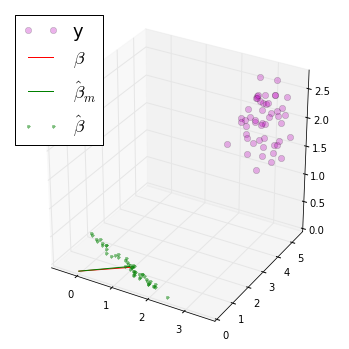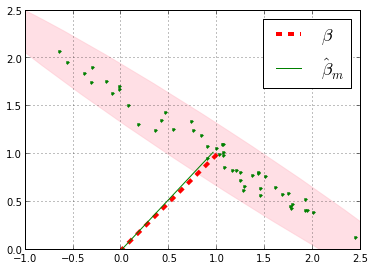## Introduction¶

In this section, we consider the famous Gauss-Markov problem which will give us an opportunity to use all the material we have so far developed. The Gauss-Markov is the fundamental model for noisy parameter estimation because it estimates unobservable parameters given a noisy indirect measurement. Incarnations of the same model appear in all studies of Gaussian models. This case is an excellent opportunity to use everything we have so far learned about projection and conditional expectation.

Following Luenberger (1997), let's consider the following problem:

$$\mathbf{y} = \mathbf{W} \boldsymbol{\beta} + \boldsymbol{\epsilon}$$

where $\mathbf{W}$ is a $n \times m$ matrix, and $\mathbf{y}$ is a $n \times 1$ vector. Also, $\boldsymbol{\epsilon}$ is a $n$-dimensional random vector with zero-mean and covariance

$$\mathbb{E}( \boldsymbol{\epsilon} \boldsymbol{\epsilon}^T) = \mathbf{Q}$$

Note that real systems usually provide a calibration mode where you can estimate $\mathbf{Q}$ so it's not fantastical to assume you have some knowledge of the noise statistics. The problem is to find a matrix $\mathbf{K}$ so that $\boldsymbol{\hat{\beta}} = \mathbf{K} \mathbf{y}$ approximates $\boldsymbol{\beta}$. Note that we only have knowledge of $\boldsymbol{\beta}$ via $\mathbf{y}$ so we can't measure it directly. Further, note that $\mathbf{K}$ is a matrix, not a vector, so there are $m \times n$ entries to compute.

We can approach this problem the usual way by trying to solve the MMSE problem:

$$\min_K \mathbb{E}(|| \boldsymbol{\hat{\beta}}- \boldsymbol{\beta} ||^2)$$

which we can write out as

$$\min_K \mathbb{E}(|| \boldsymbol{\hat{\beta}}- \boldsymbol{\beta} ||^2) = \min_K\mathbb{E}(|| \mathbf{K}\mathbf{y}- \boldsymbol{\beta} ||^2) = \min_K\mathbb{E}(|| \mathbf{K}\mathbf{W}\mathbf{\boldsymbol{\beta}}+\mathbf{K}\boldsymbol{\epsilon}- \boldsymbol{\beta} ||^2)$$

and since $\boldsymbol{\epsilon}$ is the only random variable here, this simplifies to

$$\min_K || \mathbf{K}\mathbf{W}\mathbf{\boldsymbol{\beta}}- \boldsymbol{\beta} ||^2 + \mathbb{E}(||\mathbf{K}\boldsymbol{\epsilon} ||^2)$$

The next step is to compute

$\DeclareMathOperator{\Tr}{Trace}$ $$\mathbb{E}(||\mathbf{K}\boldsymbol{\epsilon} ||^2) = \mathbb{E}(\boldsymbol{\epsilon}^T \mathbf{K}^T \mathbf{K}^T \boldsymbol{\epsilon})=\Tr(\mathbf{K \mathbb{E}(\boldsymbol{\epsilon}\boldsymbol{\epsilon}^T) K}^T)=\Tr(\mathbf{K Q K}^T)$$

using the properties of the trace of a matrix. We can assemble everything as

$$\min_K || \mathbf{K W} \boldsymbol{\beta} - \boldsymbol{\beta}||^2 + \Tr(\mathbf{K Q K}^T)$$

Now, if we were to solve this for $\mathbf{K}$, it would be a function of $\boldsymbol{\beta}$, which is the same thing as saying that the estimator, $\boldsymbol{\hat{\beta}}$, is a function of what we are trying to estimate, $\boldsymbol{\beta}$, which makes no sense. However, writing this out tells us that if we had $\mathbf{K W}= \mathbf{I}$, then the first term vanishes and the problem simplifies to

$$\min_K \Tr(\mathbf{K Q K}^T)$$

with

$$\mathbf{KW} = \mathbf{I}$$

This requirement is the same as asserting that the estimator is unbiased,

$$\mathbb{E}( \boldsymbol{\hat{\beta}}) = \mathbf{KW} \boldsymbol{\beta} = \boldsymbol{\beta}$$

To line this problem up with our earlier work, let's consider the $i^{th}$ column of $\mathbf{K}$, $\mathbf{k}_i$. Now, we can re-write the problem as

$$\min_k (\mathbf{k}_i^T \mathbf{Q} \mathbf{k}_i)$$

with

$$\mathbf{k}_i^T \mathbf{W} = \mathbf{e}_i$$

and from our previous work on contrained optimization, we know the solution to this:

$$\mathbf{k}_i = \mathbf{Q}^{-1} \mathbf{W}(\mathbf{W}^T \mathbf{Q^{-1} W})^{-1}\mathbf{e}_i$$

Now all we have to do is stack these together for the general solution:

$$\mathbf{K} = (\mathbf{W}^T \mathbf{Q^{-1} W})^{-1} \mathbf{W}^T\mathbf{Q}^{-1}$$

It's easy when you have all of the concepts lined up! For completeness, the covariance of the error is

$$\mathbb{E}(\hat{\boldsymbol{\beta}}-\boldsymbol{\beta}) (\hat{\boldsymbol{\beta}}-\boldsymbol{\beta})^T = \mathbb{E}(\mathbf{K} \boldsymbol{\epsilon} \boldsymbol{\epsilon}^T \mathbf{K}^T)=\mathbf{K}\mathbf{Q}\mathbf{K}^T =(\mathbf{W}^T \mathbf{Q}^{-1} \mathbf{W})^{-1}$$

The following simulation illustrates these results.

In :
from mpl_toolkits.mplot3d import proj3d
from numpy.linalg import inv
import matplotlib.pyplot as plt
import numpy as np
from numpy import matrix, linalg, ones, array

Q = np.eye(3)*.1 # error covariance matrix
#Q[0,0]=1

beta = matrix(ones((2,1))) # this is what we are trying estimate
W = matrix([[1,2],
[2,3],
[1,1]])

ntrials = 50
epsilon = np.random.multivariate_normal((0,0,0),Q,ntrials).T
y=W*beta+epsilon

K=inv(W.T*inv(Q)*W)*matrix(W.T)*inv(Q)
b=K*y #estimated beta from data

fig = plt.figure()
fig.set_size_inches([6,6])

# some convenience definitions for plotting
bb = array(b)
bm = bb.mean(1)
yy = array(y)

ax.plot3D(yy[0,:],yy[1,:],yy[2,:],'mo',label='y',alpha=0.3)
ax.plot3D([beta[0,0],0],[beta[1,0],0],[0,0],'r-',label=r'$\beta$')
ax.plot3D([bm,0],[bm,0],[0,0],'g-',lw=1,label=r'$\hat{\beta}_m$')
ax.plot3D(bb[0,:],bb[1,:],0*bb[1,:],'.g',alpha=0.5,lw=3,label=r'$\hat{\beta}$')
ax.legend(loc=0,fontsize=18)
plt.show()The figure above show the simulated $\mathbf{y}$ data as magenta circles. The green dots show the corresponding estimates, $\boldsymbol{\hat{\beta}}$ for each sample. The red and green lines show the true value of $\boldsymbol{\beta}$ versus the average of the estimated $\boldsymbol{\beta}$-values, $\boldsymbol{\hat{\beta_m}}$. The matrix $\mathbf{K}$ maps the magenta circles in the corresponding green dots. Note there are many possible ways to map the magenta circles to the plane, but the $\mathbf{K}$ is the ones that minimizes the MSE for $\boldsymbol{\beta}$.

The figure below shows more detail in the horizontal xy-plane above.

In :
from  matplotlib.patches import Ellipse

fig, ax = plt.subplots()
fig.set_size_inches((6,6))
ax.set_aspect(1)
ax.plot(bb[0,:],bb[1,:],'g.')
ax.plot([beta[0,0],0],[beta[1,0],0],'r--',label=r'$\beta$',lw=4.)
ax.plot([bm,0],[bm,0],'g-',lw=1,label=r'$\hat{\beta}_m$')
ax.legend(loc=0,fontsize=18)
ax.grid()

bm_cov = inv(W.T*Q*W)
U,S,V = linalg.svd(bm_cov)

err = np.sqrt((matrix(bm))*(bm_cov)*(matrix(bm).T))
theta = np.arccos(U[0,1])/np.pi*180

,angle=theta,color='pink',alpha=0.5))

plt.show()The figure above shows the green dots, which are individual estimates of $\boldsymbol{\hat{\beta}}$ from the corresponding simulated $\mathbf{y}$ data. The red dashed line is the true value for $\boldsymbol{\beta}$ and the green line ($\boldsymbol{\hat{\beta_m}}$ ) is the average of all the green dots. Note there is hardly a visual difference between them. The pink ellipse provides some scale as to the covariance of the estimated $\boldsymbol{\beta}$ values. I invite you to download this IPython notebook and tweak the values for the error covariance or any other parameter. All of the plots should update and scale correctly.

## Summary¶

The Gauss-Markov problem is the cornerstone of all Gaussian modeling and is thus one of the most powerful models used in signal processing. We showed how to estimate the unobservable parameters given noisy measurements using our previous work on projection. We also coded up a short example to illustrate how this works in a simulation. For a much more detailed approach, see Luenberger (1997).

As usual, the corresponding IPython notebook for this post is available for download here.